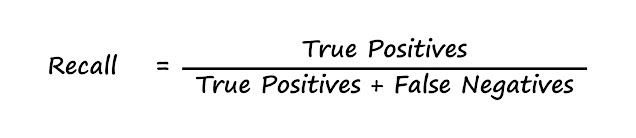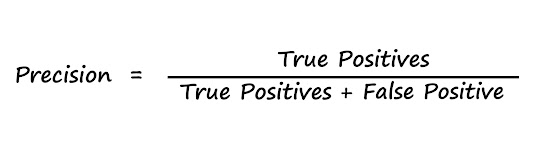# What is Precision and Recall in machine learning?

Precision and recall are the two terms which confused me a lot in my machine learning path. These terms sound easy but they are not as easy as they sound. And the high-level definition provided in most of the blogs are way out of my understanding, actually I never find those definitions easy to understand. So, I tried to find some other way to understand these terms with real world example.

So, take an example where you got an imbalanced data for skin cancer and you were asked to create a model to detect skin cancer. You created a model which detects skin cancer with very high accuracy say 98%. But, is it the perfect model? Or we can say, is accuracy is the right metric for evaluation? The answer is “No”. Because the rate of skin cancer in public is very low and that’s why we are having an imbalance dataset in the first place and just by classifying every image as “negative” means no cancer, we can achieve very good accuracy.

In case of Imbalanced dataset where one class is highly outnumbered by another class “Accuracy” is not a good metric to use. Imagine if we detect a person having a skin cancer as negative, it can cause serious problem and can become reason for the death of that person. So, in such problem we focus on positive cases and here comes in the play precision and recall.

## Recall

In technical terms recall is the ability of a model to find all the relevant cases in the dataset or in other words recall is the number of true positives divided by number of true positives plus the number of false negative.Formula for recall

Didn’t get it! Me neither. So, lets take an example. Suppose your parents give you a unique gift every year on your birthday and now its your 21st birthday and your parents ask you “do you remember all the birthday presents form us?”. So, you start recalling them one by one. Now suppose you remember 15 out of 20 correctly. Recall is the ratio of a number of events you can recall correctly. In our case you recalled 15 presents correctly. So, you recall ratio is 15/20 that is 0.75 or 75%. If you recall all the presents correctly then your recall ratio will become 1.0 or 100%.

Now let’s see how recall works in our skin cancer problem we discussed above.

If you detect every person as a cancer patient then your recall will become 1.0 or 100%. So, is it a perfect model? Again No. There is a trade-off between recall and precision. If we increase recall our precision will decrease and vice versa. Now that precision has jumped into the story lets understand precision deeply.

## Precision

Precision is defined as the number of true positives divided by the number of true positives plus the number of false positives.Formula for Precision

Got it? If not, don’t worry just hold on and keep going. Let’s take our previous example where your parents asked you to recall all the birthday presents. Suppose you recalled 20 out of 20 presents correctly but to recall all these present correctly you answered 25 times. You recalled all the present correctly but you were not precise enough. So, precision is the ratio of number of events you can correctly recall to a number all events you recall (correct and wrong both).

In above example you recalled 20 events correctly so your recall is 1.0 or 100% but to recall all events correctly you answered 25 times out of which 20 were correct and 5 were wrong. So, your precision ratio becomes 20/25 that is 0.8 or 80%.

Let’s put this knowledge in our cancer detection problem.

Suppose you detect every cancer patient correctly without making any mistake. Which means you detected only those people as cancer patients who were actually having cancer means no false positive. In such case your precision will become 1.0 or 100% but your recall will be low because there are still false negatives where you detect a healthy person as a cancer patient.

Now you might be having a question that if you cannot make neither precision nor recall as 1.0 then how you are going to evaluate your model? Remember earlier we talked about trade-off between precision and recall. To find a sweet spot between precision and recall we use some other metrics but most commonly used is the F1-score. It takes into account both precision and recall and gives a harmonic mean.F1-score

Another question you might be having is that, why we don’t just simply take the average of precision and recall? F1-score gives equal weight to both the metric. For example, if our model has a recall value of 1.0 and precision 0 then a simple average will result in 0.5 but F1-score will be 0 in this case. So higher the F1-score the better model will be.

So that’s all about the precision and recall. I hope I was able to make you understand these terms clearly.

It takes a lot of time and effort to write such articles. Please donate a small amount and help me make my living. Thank you.

Check out my other article on neural networks where I explained neural networks as simple as possible with example.